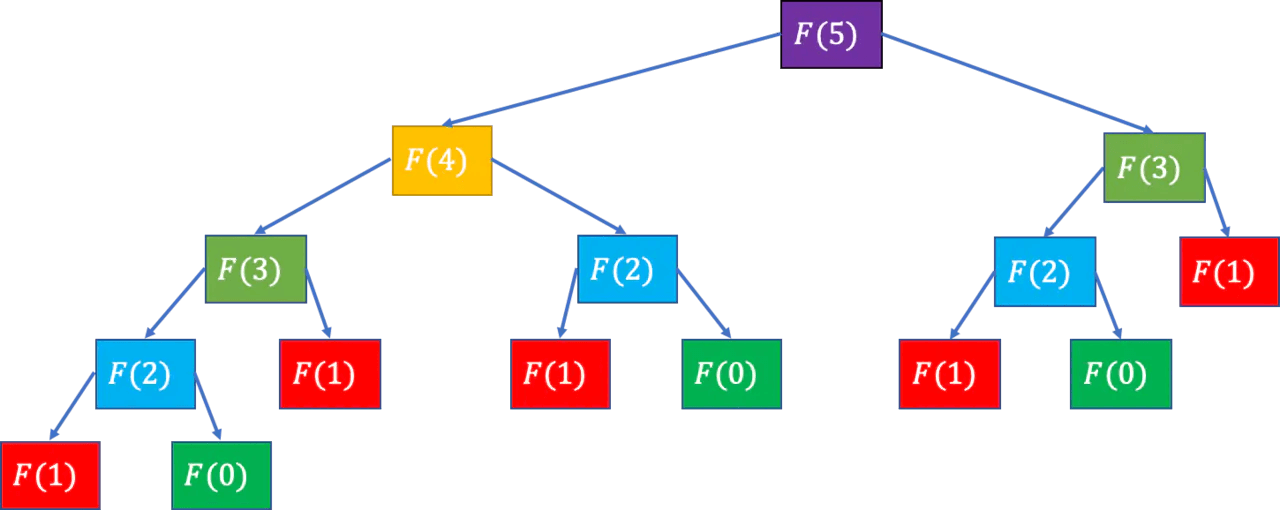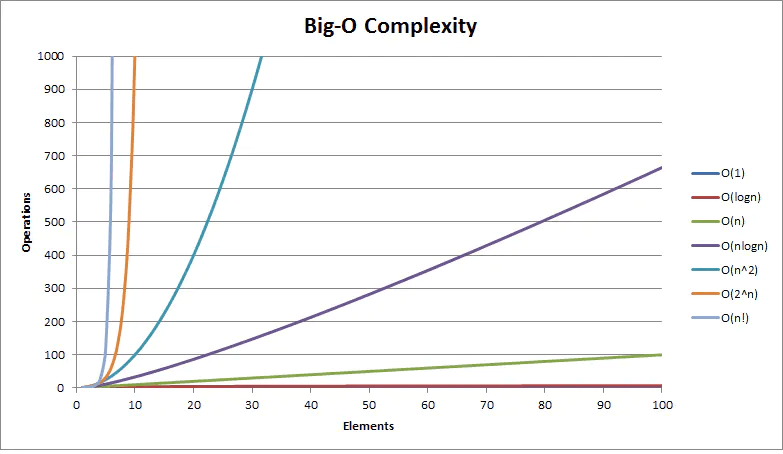# 算法复杂度

## 时间复杂度

### 常见的时间复杂度

#### 常数的时间复杂度 $O(1)$

const sum = 1 + 2

#### 线性的时间复杂度 $O(n)$

for (let i = 0; i < n; i++) {
console.log(i)
}

for 循环里面的代码会执行 $n$ 次，因此它执行所需时间是随着 $n$ 的变化而变化，因此得到复杂度为 $O(n)$。

#### 平方的时间复杂度 $O(n^2)$

for (let i = 0; i < n; i++) {
for (let j = 0; j < n; i++) {
console.log(i, j)
}
}

#### 对数的时间复杂度 $O(log(n))$

let i = 1
while(i <= y) {
i *= 2
}

$$2^1 -> 2^2 -> 2^3 -> … -> 2^x$$

$$log_c a * log_a b = log_c b$$

#### 递归的时间复杂度

$$F(0) = 1, F(1) = 1, F(n) = F(n−1) + F(n−2)(n ≥ 2, n \in N ∗)$$

function fibonacci(n) {
if (n === 0 || n === 1) return 1
return fibonacci(n - 1) + fibonacci(n - 2)
}// 黄金分割率
const goldenRatio = (1 + Math.sqrt(5)) / 2
function fibonacci(n) {
return Math.round((Math.pow(goldenRatio, n - 1) + Math.pow(goldenRatio, n)) / Math.sqrt(5))
}

$$\frac{f_{n+5}}{f_n} \approx a = \frac{1}{2}({1 + \sqrt{5}}) = \varphi \approx 1.6180339887…$$

## 空间复杂度

### $O(1)$ 复杂度

const i = 1
const j = 2

### $O(n)$ 复杂度

const arr = new Array(n)
for (let i = 0; i < n; i++) {
console.log(arr[i])
}

## 总结